# What is the least common multiple of 6 and 7?Frida

Solution:

Concept:The least common multiple of two numbers is the smallest number which is divisible by both the numbers.

Calculation:

Step:1 The LCM of two numbers are taken by factoring out the numbers into smaller values.

Step:2 The least common multiple of 6 and 7 is calculated as shown below:

Step:3 The factored form of 6 is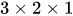and that of 7is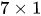.

Step:4 The common between the factorized value of 6 and 7 is taken as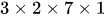.

It can also be calculated using the method below: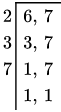Hence, the LCM is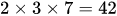.

Answer:The least common multiple of 6 and 7 is 42.

Similar Problems:

Problem 1:What is the least common multiple of 3 and 9?

Solution:

Step:1 The LCM of two numbers are taken by factoring out the numbers into smaller values.

The least common multiple of 3 and 9 is calculated as shown below:

Step:2 The factored form of 3 is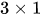and that of 9 is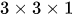.

Step:3 The common between the factorized value of 3 and 9 is taken as.

It can also be calculated using the method below: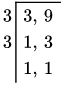Hence, the LCM is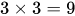.

Answer:The least common multiple of 3 and 9 is 9.

Problem 2:What is the least common multiple of 4 and 8?

Solution: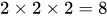Step:1 The LCM of two numbers are taken by factoring out the numbers into smaller values.

Step:2 The least common multiple of 4 and 8 is calculated as shown below:

Step:3 The factored form of 4 is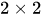and that of 8 is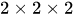.

Step:4 The common between the factorized value of 4 and 8 is taken as.

It can also be calculated using the method below: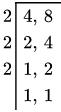Hence, the LCM is .

Answer:The least common multiple of 4 and 8 is 8.

### Need homework help now?

tutors online

Related Questions
##### (t) in °C of the pot of soup at time t (in The initial temperature of a pot of soup is 80°C. The temperature minutes) satisfies the differential equation dT di T-20 10 (a) Solve the differential equation, and find an explicit expression for the function (). By separating variables, integrating and manipulating arbitrary constants, . We are also told that so that and so . ...
Notes
Me

OR

Don't have an account?

Join OneClass

Access over 10 million pages of study
documents for 1.3 million courses.

Join to view

OR

By registering, I agree to the Terms and Privacy Policies
Just a few more details

So we can recommend you notes for your school.